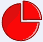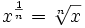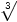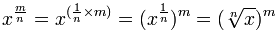# 分数指数

## 整数指数一个数的指数代表把多少个这个数乘在一起。 例子：82 = 8 × 8 = 64 用文字来表达： 82 可以叫 "8的二次方"、"8的二次幂" 或 "8的平方"

## 分数指数

 指数是1/2代表平方根 指数是1/3代表立方根 指数是1/4代表四次方根 依此类推！## 为什么？

### 例子：9½ × 9½ 是什么？

9½ × 9½ = 9(½+½) = 9(1) = 9

√9 × √9 = 9

9½ × 9½ = 9

## 用另一个分数来试试看

### 例子：16¼

16¼ ×16¼ ×16¼ ×16¼ = 16(¼+¼+¼+¼) = 16(1) = 16

，故此 16¼ 是 16 的 四次方根

## 通用规则

x1/n = x 的 n次方根分数指数，像1/n，的意思是 取 n次方根：### 例子：271/3 是什么？

1/3 =27 = 3

## 更复杂的分数呢？

• 整数部分（m），和
• 分数部分（1/n

###分数指数，像 m/n ,的意思是：   取 m 次幂，然后取 n 次方根 或 取 n 次方根，然后取 m 次幂### 例子：43/2 是什么？

43/2 = 43×(1/2) = √(43) = √(4×4×4) = √(64) = 8

43/2 = 4(1/2)×3 = (√4)3 = (2)3 = 8

### 例子：274/3 是什么？?

274/3 = 274×(1/3) =(274) =(531441) = 81

274/3 = 27(1/3)×4 = (27)4 = (3)4 = 81

## 现在来玩玩这个图！

• 从 m=1 和 n=1 开始，然后慢慢增大 n，留心看 1/2、1/3 和 1/4
• 接着以 m=2，把 n 上下改变，留心看像 2/3 等等的分数
• 尝试把指数调成 −1
• 最后试试把 m 增大，再把 n 减少；然后 减少 m，再 增大 n：曲线会来回绕圈